# Edexcel A Level Economics A:复习笔记3.3.1 Revenue

### Total, Average & Marginal Revenue

• Total revenue is the total value of all sales a firm incurs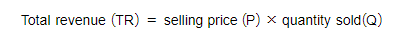• Average revenue is the overall revenue per unit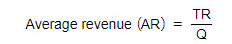• Marginal revenue is the extra revenue received from the sale of an additional unit of output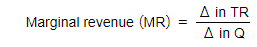• The relationship between TR, AR & MR is different in perfect competition and imperfect competition

#### Perfect Competition

The Relationship Between TR, AR & MR In Perfect Competition Can Be Seen Numerically Below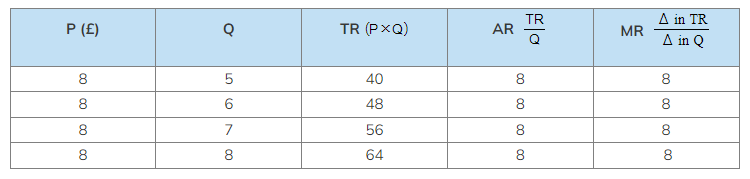• The situation in the table above is illustrated in the diagram below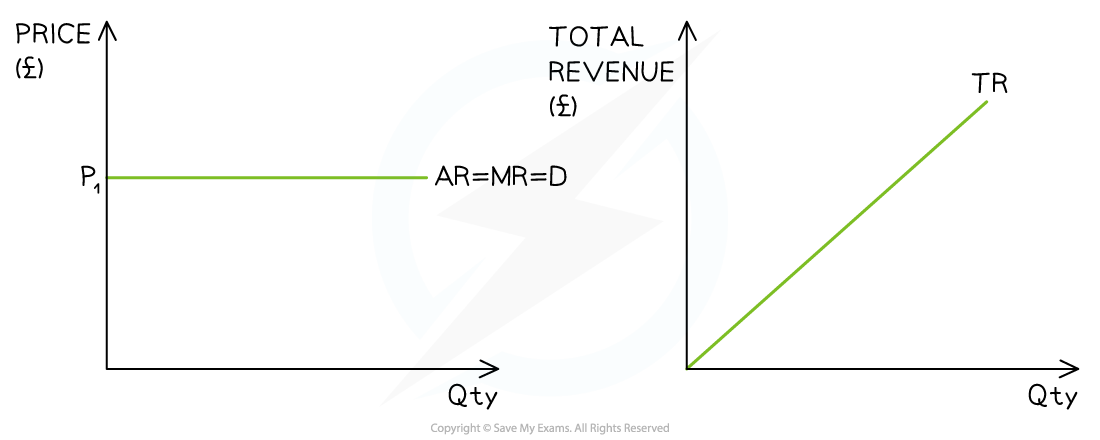An illustration of the relationship between AR, MR & TR

Observations

•  The firm is a price taker at P1 (£8)
• Every unit of output is sold at the same price
• A higher price would decrease sales to zero
• A lower price would result in all sellers lowering their price
• TR increases at a constant rate
• MR = AR = Demand

#### Imperfect Competition

The Relationship Between TR, AR & MR For Imperfect Competition Can Be Seen Numerically Below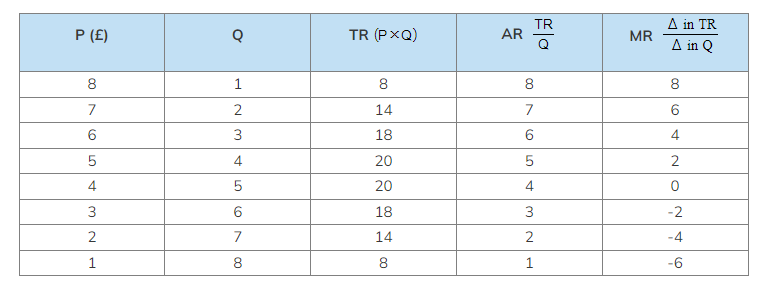• The situation in the table above is illustrated in the diagram below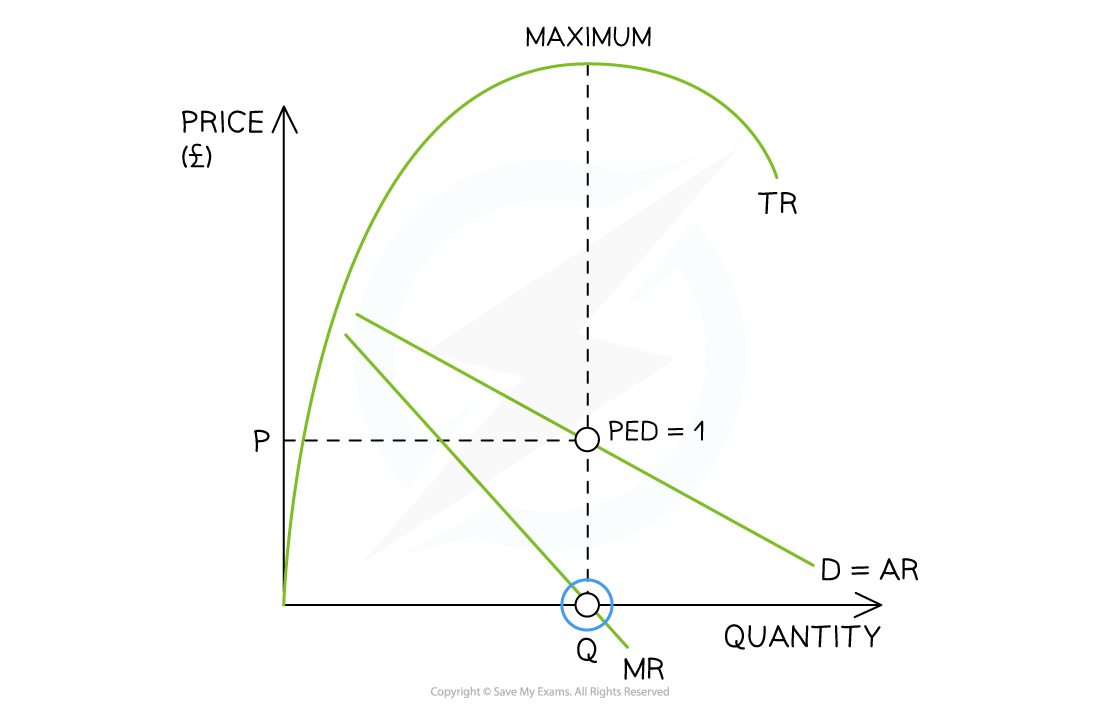An illustration of the relationship between AR, MR & TR for imperfect competition

Observations

•  The firm is a price maker
• In order to sell an additional unit of output, the price (AR) must be lowered
• Both AR & MR fall with additional units of sale
• When the AR falls, the MR falls by twice as much
• The gradient of the MR curve is twice as steep as the AR curve
• TR is maximised when MR = 0
• AR is the demand (D) curve
• When MR = 0, then the price elasticity of demand (PED) = 1
• This is unitary elasticity

### PED & Total Revenue

• The total revenue rule states that in order to maximise revenue, firms should increase the price of products that are inelastic in demand and decrease prices on products that are elastic in demand
• This can be illustrated using an average revenue (AR) curve which is the demand curve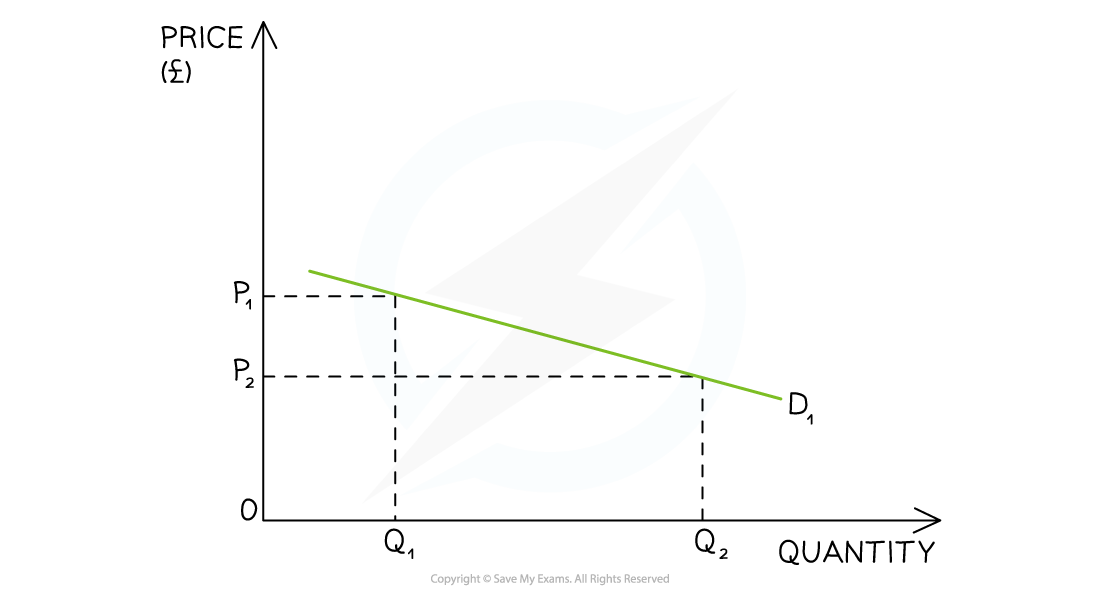An illustration of price elastic demand where a small decrease in price from P1→P2 causes a large increase in quantity demanded from Q1→ Q2

Observations

• When a good/service is price elastic in demand, there is a greater proportional increase in the quantity demanded to a decrease in price
• TR is higher once the price has been decreased
•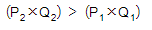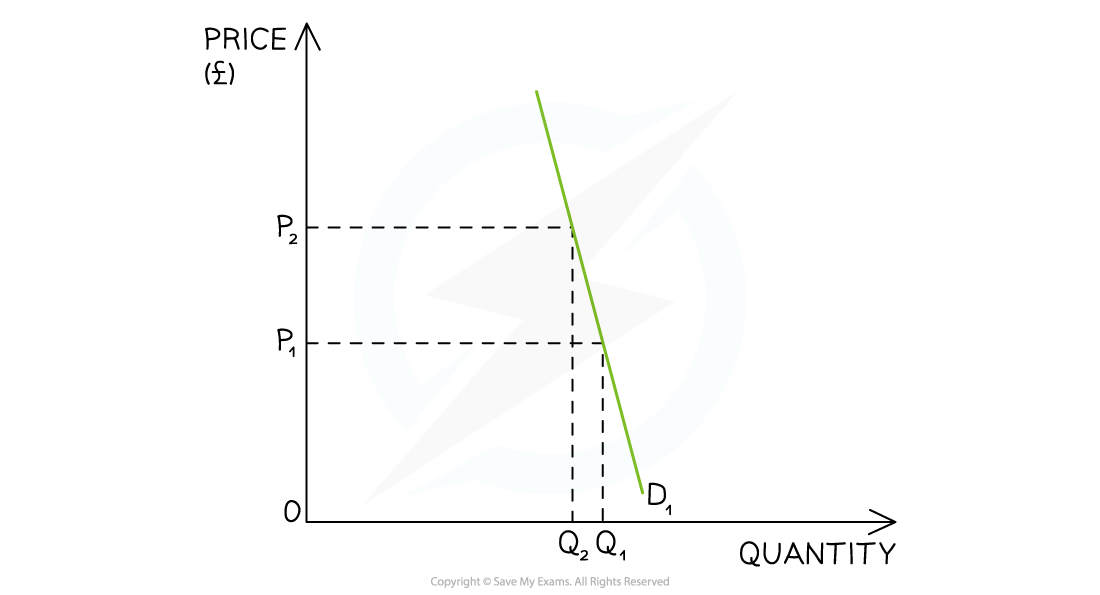An illustration of price inelastic demand where a large increase in price from P1→P2 causes a small decrease in quantity demanded from Q1→ Q2

Observations

• When a good/service is price inelastic in demand, there is a smaller than proportional decrease in the quantity demanded to an increase in price
• TR is higher once the price has been increased
•